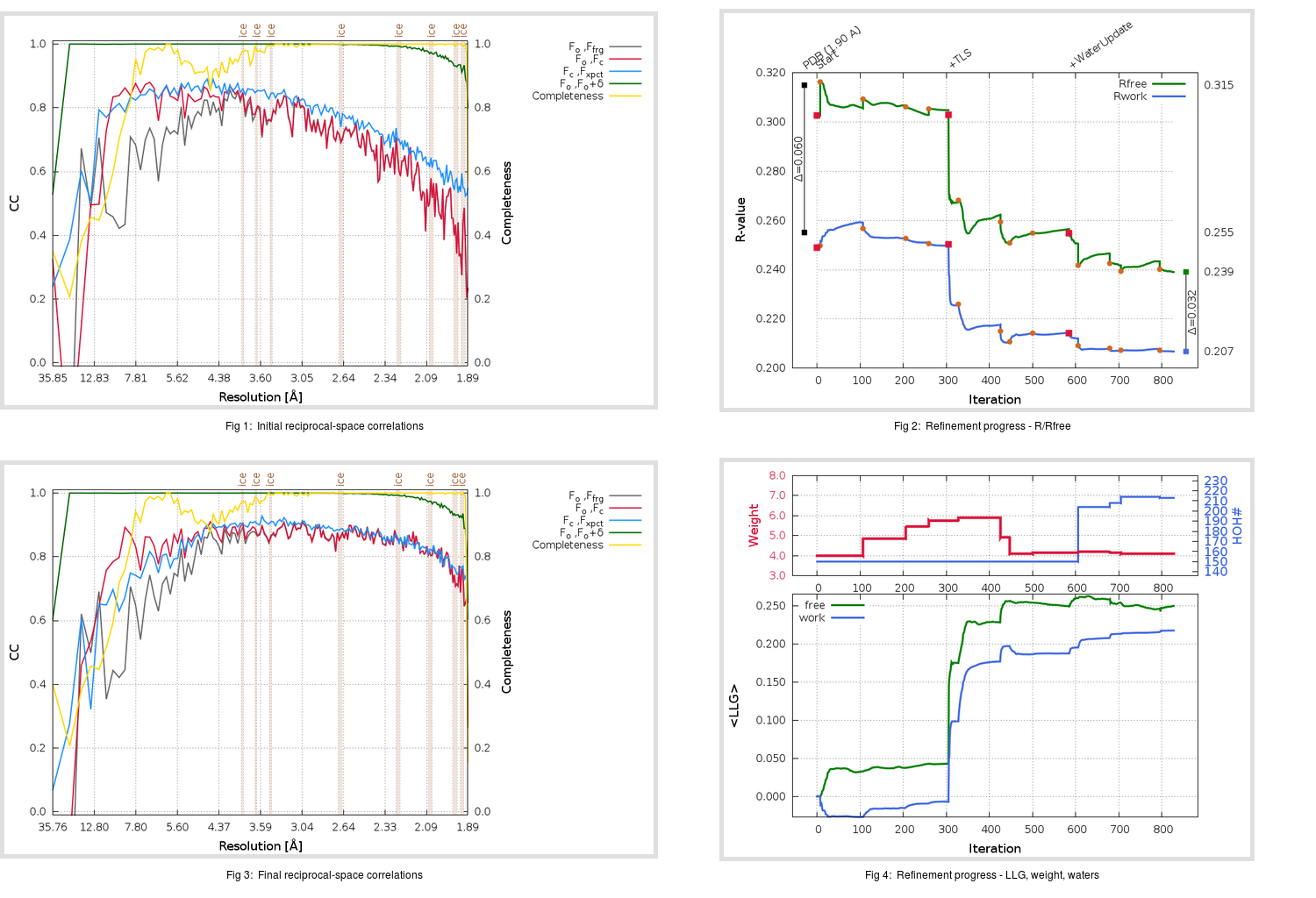Content:

```    Diffraction limits & principal axes of ellipsoid fitted to diffraction cut-off surface:
1.867         1.0000   0.0000   0.0000       0.992 a* - 0.124 c*
1.853         0.0000   1.0000   0.0000       b*
1.822         0.0000   0.0000   1.0000       c*
```

## Deposited

` `
 Date deposited Date data collection Resolution R, Rfree 20050808 20031205 1.90 0.2550 0.3150

Molprobity (CCP4 7.0 version) summary:

```Ramachandran outliers =   1.67 %
favored =  94.00 %
Rotamer outliers      =   4.14 %
C-beta deviations     =     3
Clashscore            =  20.57
RMS(bonds)            =   0.0181
RMS(angles)           =   2.04
MolProbity score      =   2.68
Resolution            =   1.90
R-work                =   0.2550
R-free                =   0.3150
```

```Number of waters      =   150

<B> (all atoms) =   51.09 ( sd =   14.80 ) for       2576 non-hydrogen atoms
<B>   (protein) =   50.93 ( sd =   15.01 ) for       2386 non-hydrogen atoms
<B>     (water) =   50.34 ( sd =    9.36 ) for        150 non-hydrogen atoms
<B>    (others) =   63.21 ( sd =   13.48 ) for         40 non-hydrogen atoms

B min/max       (all non-hydrogen atoms) =   21.71 /   99.38
B min/max   (protein non-hydrogen atoms) =   22.28 /   99.38
B min/max     (water non-hydrogen atoms) =   21.71 /   75.35
B min/max     (other non-hydrogen atoms) =   43.90 /   85.43
```

## BUSTER (re-)refinement

` `

Molprobity (CCP4 7.0 version) summary:

```Ramachandran outliers =   0.00 %
favored =  96.67 %
Rotamer outliers      =   2.26 %
C-beta deviations     =     0
Clashscore            =   2.70
RMS(bonds)            =   0.0113
RMS(angles)           =   1.56
MolProbity score      =   1.54
Resolution            =   1.89
R-work                =   0.2068
R-free                =   0.2390
```

```Number of waters      =   213

<B> (all atoms) =   56.88 ( sd =   17.59 ) for       2639 non-hydrogen atoms
<B>   (protein) =   56.55 ( sd =   18.00 ) for       2386 non-hydrogen atoms
<B>     (water) =   60.19 ( sd =   13.22 ) for        213 non-hydrogen atoms
<B>    (others) =   58.80 ( sd =    9.75 ) for         40 non-hydrogen atoms

B min/max       (all non-hydrogen atoms) =   30.45 /  113.84
B min/max   (protein non-hydrogen atoms) =   30.45 /  113.84
B min/max     (water non-hydrogen atoms) =   32.05 /  104.60
B min/max     (other non-hydrogen atoms) =   44.75 /   74.96
```

Refinement progression:Results:

` `
 File Remark 2ALV_aB_refine.01_03_refine.pdb.gz exact refinement commands are in header 2ALV_aB_refine.01_03_refine.mtz.gz including original deposited data and several re-refinement map coefficients 2ALV_aB_refine.01_03_BUSTER_model.cif.gz including any non-standard compound restraints 2ALV_aB_refine.01_03_BUSTER_refln.cif.gz